### ICSE Class 10 Maths Previous Year Question Paper 2018# ICSE CLASS 10 MATHS PREVIOUS YEAR PAPER 2018

ICSE – Class 10th

Previous Year – 2018

Mathematics

Time: 2 ½ Hrs                                                                                                                               Marks:80

General Instructions:-

•  Attempt all questions from Section A and any four questions from Section B.
•  All working, including rough work, must be clearly shown and must be done on the same sheet as the rest of the answer.
•  Omission of essential working will result in loss of marks.
•  The intended marks for questions or parts of questions are given in brackets [].
• Mathematical tables are provided.

SECTION A (40 Marks)

Attempt all questions from this Section.

1.

a)

Find the value of and ‘y’ if:

$2left[begin{array}{cc}x & 7 \ 9 & y-5end{array}right]+left[begin{array}{rr}6 & -7 \ 4 & 5end{array}right]=left[begin{array}{cc}10 & 7 \ 22 & 15end{array}right]$

3

b)

Sonia had a recurring deposit account in a bank and deposited Rs. 600 per month for $2^{1} / 2$ years. If the rate of interest was $10 %$ p.a., find the maturity value of this account.

3

c)

Cards bearing numbers $2,4,6,8,10,12,14,16,18$ and 20 are kept in a bag. A card is drawn at random from the bag. Find the probability of getting a card which is:

4

i.

a prime number.

ii.

a number divisible by 4.

iii.

a number that is a multiple of 6.

iv.

an odd number.

2.

a)

The circumference of the base of a cylindrical vessel is $132 mathrm{~cm}$ and its height is 25 $mathrm{cm}$. Find the

3

i.

ii.

volume of cylinder (use $pi=frac{22}{7}$ )

b)

If $(mathrm{k}-3),(2 mathrm{k}+1)$ and $(4 mathrm{k}+3)$ are three consecutive terms of an A.P., find the value of $mathrm{k}$.

3

c)

PQRS is a cyclic quadrilateral. Given $angle mathrm{QPS}=73^{circ}, angle mathrm{PQS}=55^{circ}$ and $angle mathrm{PSR}=82^{circ}$,

calculate: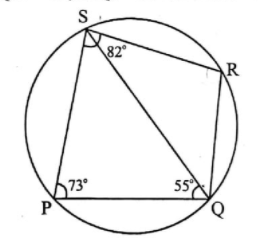4

i.

$angle QRS$

ii.

$angle RQS$

iii.

$angle PRQ$

3.

a)

If $(x+2)$ and $(x+3)$ are factors of $x^{3}+a x+b$, find the values of ‘a’ and ‘b’.

3

b)

Prove that $sqrt{sec ^{2} theta+operatorname{cosec}^{2} theta}=tan theta+cot theta$

3

c)

Using a graph paper draw a histogram for the given distribution showing the number of runs scored by 50 batsmen. Estimate the mode of the data:

 Runs scored 3000- 4000 4000- 5000 5000- 6000 6000- 7000 7000- 8000 8000- 9000 9000- 10000 No. of Batsman 4 18 9 6 7 2 4

4

4.

a)

Solve the following inequation, write down the solution set and represent it on the real number line:

$-2+10 x leq 13 x+10<24+10 x, x in Z$

3

b)

If the straight lines $3 x-5 y=7$ and $4 x+a y+9=0$ are perpendicular to one another, find the value of a.

3

c)

Solve $x^{2}+7 x=7$ and give your answer correct to two decimal places.

4

SECTION B (40 Marks)

Attempt any four questions from this Section

5.

a)

The $4^{text {th }}$ term of a G.P. is 16 and the $7^{text {th }}$ term is $128 .$ Find the first term and common ratio of the series.

3

b)

A man invests Rs. 22,500 in Rs. 50 shares available at $10 %$ discount. If the dividend paid by the company is $12 %$, calculate:

3

i.

The number of shares purchased

ii.

iii.

The rate of return he gets on his investment. Give your answer correct to the nearest whole number.

c)

Use graph paper for this question (Take $2 mathrm{~cm}=1$ unit along both $x$ and y axis). $A B C D$ is a quadrilateral whose vertices are $mathrm{A}(2,2), mathrm{B}(2,-2), mathrm{C}(0,-1)$ and $mathrm{D}(0,1)$.

4

i.

Reflect quadrilateral $mathrm{ABCD}$ on the $mathrm{y}$ -axis and name it as A’B’CD.

ii.

Write down the coordinates of $mathrm{A}^{prime}$ and $mathrm{B}^{prime}$.

iii.

Name two points which are invariant under the above reflection.

iv.

Name the polygon $mathrm{A}^{prime} mathrm{B}^{prime} mathrm{CD}$.

6.

a)

Using properties of proportion, solve for $x$. Given that $x$ is positive:

$frac{2 x+sqrt{4 x^{2}-1}}{2 x-sqrt{4 x^{2}-1}}=4$

3

b)

if $A=left[begin{array}{ll}2 & 3 \ 5 & 7end{array}right], B=left[begin{array}{rr}0 & 4 \ -1 & 7end{array}right]$ and $c=left[begin{array}{rr}1 & 0 \ -1 & 4end{array}right]$, find $A C+B^{2}-10 C .$

3

c)

Prove that $(1+cot theta-operatorname{cosec} theta)(1+tan theta+sec theta)=2$

4

7.

a)

Find the value of $mathrm{k}$ for which the following equation has equal roots. $x^{2}+4 k x+left(k^{2}-k+2right)=0$

3

b)

On a map drawn to a scale of $1: 50,000$, a rectangular plot of land ABCD has the following dimensions. $mathrm{AB}=6 mathrm{~cm} ; mathrm{BC}=8 mathrm{~cm}$ and all angles are right angles. Find: $$

3

i.

the actual length of the diagonal distance AC of the plot in $mathrm{km}$.

ii.

the actual area of the plot in sq. $mathrm{km}$.

c)

$mathrm{A}(2,5), mathrm{B}(-1,2)$ and $mathrm{C}(5,8)$ are the vertices of a triangle $mathrm{ABC},{ }^{prime} mathrm{M}^{prime}$ is a point on $mathrm{AB}$

such that $mathrm{AM}: mathrm{MB}=1: 2$. Find the co-ordinates of ‘ $mathrm{M}$ ‘. Hence find the equation of the line passing through the points $mathrm{C}$ and $mathrm{M}$.

4

8.

a)

Rs. 7500 were divided equally among a certain number of children. Had there been 20 less children, each would have received Rs. 100 more. Find the original number of children.

3

b)

If the mean of the following distribution is 24 , find the value of ‘ $a$ ‘

 Marks 0-10 10-20 20-30 30-40 40-50 No. of students 7 a 8 10 5

3

c)

Using ruler and compass only, construct a $triangle mathrm{ABC}$ such that $mathrm{BC}=5 mathrm{~cm}$ and $mathrm{AB}=6.5$ $mathrm{cm}$ and $angle mathrm{ABC}=120^{circ}$

4

i.

Construct a circum-circle of $Delta mathrm{ABC}$

ii.

Construct a cyclic quadrilateral $A B C D$, such that $D$ is equidistant from $A B$ and $mathrm{BC}$.

9.

a)

Priyanka has a recurring deposit account of Rs. 1000 per month at $10 %$ per annum. If she gets Rs. 5550 as interest at the time of maturity, find the total time for which the account was held.

3

b)

In $Delta mathrm{PQR}, mathrm{MN}$ is parrallel to $mathrm{QR}$ and $frac{mathrm{PM}}{mathrm{MQ}}=frac{2}{3}$

3

i.

Find $frac{mathrm{MN}}{mathrm{QR}}$

ii.

Prove that $Delta 0 M N$ and $Delta O R Q$ are similar.

iii.

Find, Area of $Delta 0 mathrm{MN}:$ Area of $Delta mathrm{ORQ}$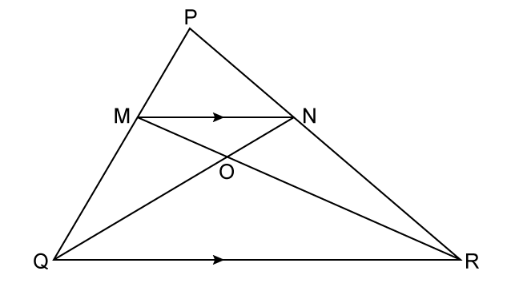c)

The following figure represents a solid consisting of a right circular cylinder with a hemisphere at one end and a cone at the other. Their common radius is $7 mathrm{~cm}$. The height of the cylinder and cone are each of $4 mathrm{~cm}$. Find the volume of the solid.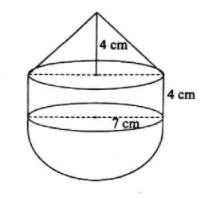4

10.

a)

Use Remainder theorem to factorize the following polynomial:

$2 x^{3}+3 x^{2}-9 x-10$

3

b)

In the figure given below ‘ 0 ‘ is the centre of the circle. If $Q R=0 P$ and $angle O R P=20^{circ}$. Find the value of ‘x’ giving reasons.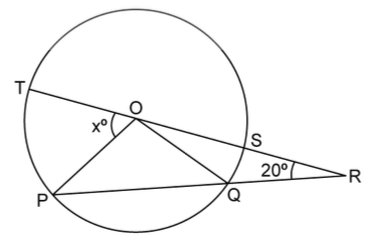3

c)

The angle of elevation from a point $mathrm{P}$ of the top of a tower $mathrm{QR}, 50 mathrm{~m}$ high is $60^{circ}$ and that of the tower PT from a point $Q$ is $30^{circ} .$ Find the height of the tower $P T$, correct to the nearest metre.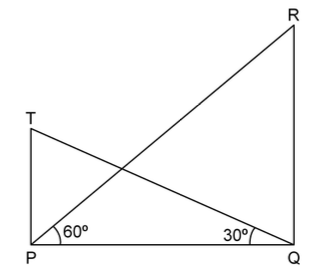4

11.

a)

The $4^{text {th }}$ term of an A.P. is 22 and $15^{text {th }}$ term is 66 . Find the first terns and the common difference. Hence find the sum of the series to 8 terms.

4

b)

Use Graph paper for this question.

A survey regarding height (in $mathrm{cm}$ ) of 60 boys belonging to Class 10 of a school was conducted. The following data was recorded:

 Height in cm. 135-140 140-145 145-150 150-155 155-160 160-165 165-170 No. of boys 4 8 20 14 7 6 1

Taking $2 mathrm{~cm}=$ height of $10 mathrm{~cm}$ along one axis and $2 mathrm{~cm}=10$ boys along the other axis draw an ogive of the above distribution. Use the graph to estimate the following:

6

i.

the median

ii.

lower Quartile

iii.

if above $158 mathrm{~cm}$ is considered as the tall boys of the class. Find the number of boys in the class who are tall.

Privacy Settings